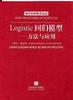logistic回归模型 订阅
《Logistic回归模型:方法与应用》是2001年高等教育出版社出版的图书，作者是王济川。 展开全文《Logistic回归模型:方法与应用》是2001年高等教育出版社出版的图书，作者是王济川。

38.20元

Logistic回归模型:方法与应用

2001-9

ISBN
9787040099102

272
Logistic回归模型内容简介
(logistic regression)属于概率型非线性回归，它是研究二分类观察结果与一些影响因素之间关系的一种多变量分析方法。在流行病学研究中，经常需要分析疾病与各危险因素之间的定量关系，为了正确说明这种关系，需要排除一些混杂因素的影响。传统上常常使用Mantel-Haenszel分层分析方法，但这一方法适用于样本含量大，分析因素较少的情况。如果用线性回归分析，由于应变量Y是一个二值变量（通常取值1或0），不满足应用条件，尤其当各因素都处于低水平或高水平时，预测值Y值可能超出0～1范围，出现不合理都现象。用logistic回归分析则可以较好的解决上述问题。在社会科学诸如社会学、心理学、人口学、政治学、经济学以及公共卫生学当中，大量的观测因变量是二分类测量。本书专题介绍了在分析二分类因变量时最常使用的统计分析模型之一——Logistic回归模型。本书深入浅出，理论联系实际，通过例题分析，并结合计算机统计软件的应用，详细介绍、阐述了该模型及其应用。同时，还介绍了如何将Logistic回归模型扩展到序次Logistic回归模型和多项Logit模型，以分析序次变量和多分类名义变量为因变量的数据。本书提供用SAS和SPSS进行具体例题分析的计算机程序及相关数据，并对这两种软件的模型估计结果进行详尽的解释和对比分析。本书的读者对象为社会科学各专业的教师及研究生，以及社会科学专业研究人员。

• ## logistic回归模型

千次阅读 多人点赞 2020-04-13 17:44:45
logistic回归模型 从这一期开始，我们准备介绍一系列机器学习算法模型，主要包括logistic回归，决策树，随机森林，关联规则，朴素贝叶斯，支持向量机模型，隐式马尔可夫模型，因子分析，主成分分析，聚类，多元线性...
logistic回归模型前言logistic回归模型logit变换几率logistic模型二项逻辑回归模型损失函数logistic回归模型的应用logistic回归模型的评价
前言
从这一期开始，我们准备介绍一系列机器学习算法模型，主要包括logistic回归，决策树，随机森林，关联规则，朴素贝叶斯，支持向量机，隐式马尔可夫，因子分析，主成分分析，聚类，多元线性回归，时间序列，协同过滤，XGBoost，LightGBM，神经网络等，一般会涵盖模型的引入背景，背后数学原理，模型的应用范围，模型优缺点及改进建议，具体工程实践。既适合刚入门机器学习的新手，也适合有一定基础的读者想要进一步掌握模型的核心要义，其中不免会涵盖许多数学符号和公式以及推导过程，如果你觉得晦涩难懂，可以来微信公众号“三行科创”交流群和大家一起讨论。
logistic回归模型
逻辑回归（Logistic Regression）是机器学习中最常见的一种用于二分类的算法模型，由于其数学原理简单易懂，作用高效，其实际应用非常广泛。虽然带回归二字，实则是分类模型，下面从logit变换开始。
logit变换
我们在研究某一结果$y$与一系列因素$(x_1,x_2,\cdots,x_n)$之间的关系的时候，最直白的想法是建立因变量和自变量的多元线性关系
$y=\theta_0+\theta_1x_1+\theta_2x_2+\cdots +\theta_nx_n$
其中  $(\theta_0,\theta_1,\theta_2,\cdots,\theta_n)$ 为模型的参数，如果因变量是数值型的话，可以解释成某某因素$x_i$变化了多少导致结果 $y$ 发生了多少变化，如果因变量 $y$ 是用来刻画结果是否（0-1）发生？或者更一般的来刻画某特定结果发生的概率（0~1）呢？这时候因素 $x_i$ 变化导致结果 $y$ 的变化恐怕微乎其微，有时候甚至忽略不计。然而实际生活中，我们知道某些关键因素会直接导致某一结果的发生，如亚马逊雨林一只蝴蝶偶尔振动翅膀，就会引起两周后美国德克萨斯州的一场龙卷风。于是，我们需要让不显著的线性关系变得显著，使得模型能够很好解释随因素的变化，结果也会发生较显著的变化，这时候，人们想到了logit变换，下图是对数函数图像从对数函数的图像来看，其在$(0,1)$之间的因变量的变化是很迅速的，也就是说自变量的微小变化会导致因变量的巨大变化，这就符合了之前想要的效果。于是，对因变量进行对数变换，右边依然保持线性关系，有下面式子
$log(y)=\theta_0+\theta_1x_1+\theta_2x_2+\cdots +\theta_nx_n$
虽然上式解决了因变量随自变量变化的敏感性问题，同时也约束了$y$的取值范围为$(0,+\infty)$。我们知道概率是用来描述某件事发生的可能性，一件事情发生与否，更应该是调和对称的，也就是说该事件发生与不发生有对立性，结果可以走向必然发生（概率为1），也可以走向必然不发生（概率为0），概率的取值范围为$(0,1)$，而等式左边 $y$ 的取值范围是$(0,+\infty)$，所以需要进一步压缩，又引进了几率。
几率
几率（odd)是指事件发生的概率与不发生的概率之比，假设事件 A 发生的概率为 $p$，不发生的概率为 $1-p$，那么事件 A 的几率为
$odd(A）=\frac{p}{1-p}$
几率恰好反应了某一事件两个对立面，具有很好的对称性，下面我们再来看一下概率和几率的关系首先，我们看到概率从0.01不断增大到 0.99，几率也从0.01随之不断变大到99，两者具有很好的正相关系，我们再对 $p$ 向两端取极限有
$\lim\limits _ {p \to 0^+}(\frac{p}{1-p})=0$
$\lim\limits _ {p \to 1^-}(\frac{p}{1-p})=+\infty$
于是，几率的取值范围就在$(0，+\infty)$，这符合我们之前的因变量取值范围的假设。
logistic模型
正因为概率和几率有如此密切对等关系，于是想能不能用几率来代替概率刻画结果发生的可能性大小，这样既能满足结果对特定因素的敏感性，又能满足对称性 ，便有了下面式子
$log(\frac{p}{1-p}) =\theta_0+ \theta_1x_1+\theta_2x_2+\cdots +\theta_nx_n$
现在，我们稍微改一改，让等式左边对数变成自然对数 $ln=log_e$，等式右边改成向量乘积形式，便有
$ln(\frac{p}{1-p})=\theta X$
其中 $\theta=(1,\theta_1,\theta_2,\cdots,\theta_n)$，$X=(1,x_1,x_2,\cdots,x_n)^T$，我们解得
$p=\frac{e^{\theta X}} {1+ e^{\theta X}}$
其中 $e$ 是自然常数，保留5位小数是2.71828。这就是我们常见的logistic模型表达式，作出其函数图像如下我们看到logistic函数图像是一条S型曲线，又名sigmoid曲线，以 $(0,0.5)$ 为对称中心，随着自变量 $x$ 不断增大，其函数值不断增大接近1，随自变量 $x$ 不断减小，其函数值不断降低接近0，函数的取值范围在 $(0,1)$ 之间，且函数曲线在中心位置变化速度最快，在两端的变化速率较慢。
从上面的操作，我们可以看到逻辑回归模型从最初的线性回归模型基础上对因变量进行 logit 变换，使得因变量对自变量显著，同时约束因变量取值范围为0到正无穷大，然后用概率表示几率，最后求出概率关于自变量的表达式，把线性回归的结果压缩在 $(0,1)$ 范围内，这样最后计算出的结果是一个0到1之间的概率值，表示某事件发生的可能性大小，可以做概率建模，这也是为什么逻辑回归叫逻辑回归，而不叫逻辑分类了。
二项逻辑回归模型
既然logistic回归把结果压缩到连续的区间 $(0,1)$，而不是离散的 0 或者1，然后我们可以取定一个阈值，通常以0.5为阈值，通过对比概率值与阈值的大小关系而定，如果计算出来的概率大于0.5，则将结果归为一类 （1），如果计算出来的概率小于0.5，则将结果归为另一类 （0），用分段函数写出来便是
$y= \begin{cases} 1&\text{p > 0.5} \\ 0 & \text{p < 0.5} \end{cases}$
这样逻辑回归就可以用来进行2分类了，在本文中，不特别指出，均指二项逻辑回归，假设数据结构如下

样本
$x_1$
$x_2$
…
$x_n$
$y$

1
$x_{11}$
$x_{12}$
…
$x_{1n}$
$y_1$

2
$x_{21}$
$x_{22}$
…
$x_{2n}$
$y_2$

$\vdots$
$\vdots$
$\vdots$
…
$\vdots$
$\vdots$

m
$x_{m1}$
$x_{m2}$
…
$x_{mn}$
$y_m$

其中 $m$表示样本个数，$n$表示影响因数的个数，$y_i(i=0,1,\cdots,m)$ 取 0 或者 1。现在我们结合数据，利用条件概率分布模型给出基于概率的二项logistic模型如下
$p(y=1|X;\theta)=\frac{e^{\theta X}}{1+e^{\theta X}}$
$p(y=0|X;\theta)=\frac{1}{1+e^{\theta X}}$
其中，$X$ 表示自变量，$y$ 表示因变量所属的类别，$\theta$ 为模型待求的参数，模型解释为在特定的因素下，模型结果取1的概率和取0的概率。模型建好了，接下来就需要进行机器训练，而怎么来给训练一种恰当反馈呢？答案是损失函数，通过损失函数来评估模型学习的好坏和改进机制。
损失函数
由前面阈值的取定原则，我们知道相当于我们用一个类别值代替概率值，而类别值是sigmoid 函数的两个最值，概率不可能时时刻刻都取到最值，这势必会造成误差，我们把这种误差称为损失，为了给出损失函数表达式，我们假设模型第 $i$ 个样本所求的概率值为 $p_i$，而真实类别值可能是 0 或者 1。
当类别真实值是 1 的情况下，所求的概率值 $p_i$ 越小，越接近0，被划为类别 0 的可能性越大，被划为类别 1 的可能性越小，导致的损失越大。反之，所求的概率值 $p_i$ 越大，越接近1，被划为类别 1 的可能性越大，被划为类别 0 的可能性越小，导致的损失越小。我们用下面的函数来描述这种变化关系
$-log p_i$
其中 $p_i\in(0,1)$ ，其图像大致如下当类别真实值是0的情况下，所求的概率值 $p_i$ 越大，越接近1，其结果的类别判定更偏向于1，导致的损失越大。反之，所求的概率值 $p_i$ 越小，越接近0，其结果的类别判断更偏向于 0，导致的损失越小。我们用下面的函数来描述这种变化关系
$-log(1-p_i)$现在就要把两种情况结合起来，就不需要分真实值是 1 还是 0 两种情况讨论，求出其期望值，做成一个交叉熵(cross entropy)的整体损失函数如下
$cost_i = -y_ilog(p_i)-(1-y_i)log(1-p_i)$
其中 $y_i$ 表示第 $i$ 个样本的真实值，$p_i$ 是根据模型计算出来的概率值，当$y_i=1$ 时，$cost_i= -log(p_i)$，当$y_i=0$时，$cost_i = -log(1-p_i)$，这符合前面两种情况。
假设现在有 $m$ 个样本，总体的损失函数为
\begin{aligned} Cost &= \sum\limits_{i=1}^m cost_i \\ &=\sum\limits_{i=1}^m[-y_ilog(p_i)-(1-y_i)log(1-p_i)]\\ \end{aligned}
代入 $p_i=\frac{e^{\theta X}}{1+e^{\theta X}}$ 得
\begin{aligned} Cost &=\sum\limits_{i=1}^m[-y_ilog(\frac{e^{\theta X}}{1+e^{\theta X}})-(1-y_i)log(1-\frac{e^{\theta X}}{1+e^{\theta X}})] \end{aligned}
上式就是二项逻辑回归的损失函数，是一个关于参数 $\theta$ 和 $X$的二元函数，也叫对数似然函数，现在问题转化为以对数似然函数为目标函数的最优化问题，其中 $\theta$ 为模型待求的参数，为了求参数 $\theta$ ，可以对目标函数求偏导数，记
$\mathcal{L}(X|\theta)=\sum\limits_{i=1}^m[-ylog(\frac{e^{\theta X}}{1+e^{\theta X}})-(1-y)log(1-\frac{e^{\theta X}}{1+e^{\theta X}})]$
对 $\mathcal{L} (X|\theta)$ 求关于 $\theta$ 的偏导，主要是里面对数函数关于 $\theta$ 的偏导数求解
\begin{aligned} &\frac{\partial \mathcal{L}(X|\theta)}{ \partial \theta} \\&=\frac{ \partial \{\sum\limits_{i=1}^m[-y_ilog(\frac{e^{\theta X}}{1+e^{\theta X}})-(1-y_i)log(1-\frac{e^{\theta X}}{1+e^{\theta X}})]\}}{\partial \theta}\\ &= \sum\limits_{i=1}^m[(-y_i)\frac{\partial log(\frac{e^{\theta X}}{1+e^{\theta X}})}{\partial \theta}-(1-y_i)\frac{\partial log(1-\frac{e^{\theta X}}{1+e^{\theta X}})}{\partial \theta}]\\ &= \sum\limits_{i=1}^m [ (-y_i)\frac{cX}{1+e^{\theta X}}-(1-y_i)(-\frac{cXe^{\theta X}}{1+e^{\theta X}})] \end{aligned}
其中 $c=ln a$，$a$ 为对数底数，令 $\frac{\partial \mathcal{L}((X|\theta)}{ \partial \theta}=0$，求出 $\theta$ 值，然后带入模型便是学到的logistic模型，假设 $\hat{\theta}$ 是上式子所求的值，那么原二项logistic模型方程为
$p(y=1|X,\hat{\theta})=\frac{e^{\hat{\theta} X}}{1+e^{\hat{\theta} X}}$
$p(y=0|X,\hat{\theta})=\frac{1}{1+e^{\hat{\theta} X}}$
logistic回归模型的应用
既然logistic回归主要用来二分类，那么凡是涉及到二分类的场景都有logistic回归的用武之地，同时，我们还可以用logistic回归模型预测某件事情的发生概率，我们常见的logistic回归模型的应用场景有
(1) 在P2P，汽车金融等领域，根据申请人的提供的资料，预测其违约的可能性大小，进而决定是否给其贷款。
(2) 电商平台根据用于购买记录预测用户下一次是否会购买某件商品。
(3) 天涯，bbs，微博，豆瓣短评等舆论平台做情感分类器。如根据某网友对某些特定主题的历史评论数据，预测其下次对某类型的主题是否会给出正面的评论。
(4) 在医疗领域，根据病人症状，预测其肿瘤是良性的还是恶性的。
(5) 根据CT，流行病学，旅行史，检测试剂结果等特点预测某位疑似病人是否真感染新型冠状病毒。
(6) 在精准营销领域，预测某个产品的收益。
logistic回归模型的评价
从logistic回归的数学原理，在阈值取定的时候一刀切的做法上面，我们能够根据logistic回归的这些特点给出模型优缺点评价
优点：

原理简单，模型清晰，操作高效，背后的概率的推导过程经得住推敲，在研究中，通常以 Logistic 回归模型作为基准，再尝试使用更复杂的算法，可以在大数据场景中使用。
使用online learning的方式更新轻松更新参数，不需要重新训练整个模型
基于概率建模，输出值落在0到1之间，并且有概率意义
求出来的参数 $\theta_i$ 代表每个特征对输出的影响，可解释性强
解决过拟合的方法很多，如L1、L2正则化，L2正则化就可以解决多重共线性问题

缺点：

对数据依赖性强，很多时候需要做特征工程，且主要用来解决线性可分问题
因为它本质上是一个线性的分类器，所以处理不好特征之间相关的情况，对模型中自变量多重共线性较为敏感，例如两个高度相关自变量同时放入模型，可能导致较弱的一个自变量回归符号不符合预期，符号被扭转，正好变负号。
logit变换过程是非线性的，在两端随着变化率微乎其微，而中间的变化很大，很敏感。 导致很多区间的变量变化对目标概率的影响没有区分度，很难确定阀值。
当特征空间很大时，性能不好
容易欠拟合，精度不高

下一期，我们将用一个实例来一睹logistic魅力。
参考文献
1，对数函数
2，python绘制对数函数
3，如何理解logistic函数
4，logit究竟是个啥？
5，逻辑回归
6，从原理到代码，轻松深入逻辑回归
7，https://www.zybuluo.com/frank-shaw/note/143260展开全文损失函数 适用范围
• ## Logistic回归模型

千次阅读 2020-04-03 12:06:39
为什么会用到logistic回归模型 普通线性回归模型的一个假设条件是当自变量取值确定时，因变量服从方差不变的正态分布。而如果因变量是类别变量，则该假设条件无法满足。为了通过自变量的线性组合来预测类别因变量的...
为什么会用到logistic回归模型
普通线性回归模型的一个假设条件是当自变量取值确定时，因变量服从方差不变的正态分布。而如果因变量是类别变量，则该假设条件无法满足。为了通过自变量的线性组合来预测类别因变量的取值，logistic回归模型应运而生。logistic回归模型可分为二分类和多分类模型。
二分类logistic回归模型
当因变量只有0，1两种取值时，因变量服从伯努利分布：预测自变量取固定值时y的取值，实际上就是要预测自变量取固定值时y所服从的伯努利分布的p值，即y=1的概率值。因为p的取值范围为[0,1]，所以p作为因变量的函数值域应为[0,1].logistic函数符合这一要求。
logistic函数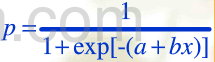函数性质：

函数取值在（0，1）内，上限p=1，下限p=0是它的两条水平渐进馅
b>0时单调递增，b<0时单调递减
x=-a/b时，p=0.5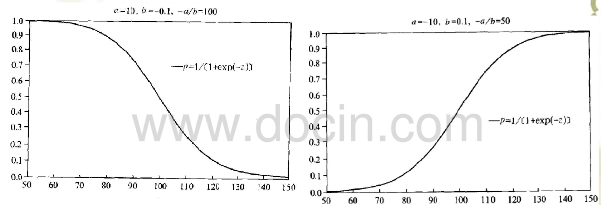因此利用logistic函数构建用于预测p的回归模型。

logistic回归模型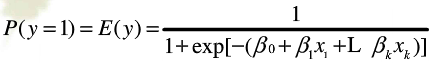其他形式：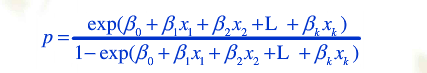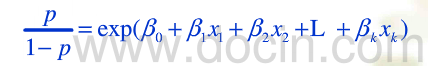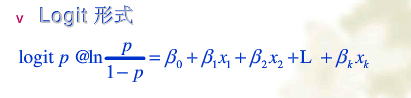logistic回归系数的意义
1.发生比(odds)2. 发生比率（odds ratio OR 或称相对风险比）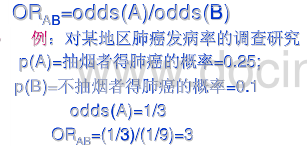3. 变量回归系数的意义
当其他的解释变量保持不变时，解释变量x2每变化一个单位，即x2+1时，原来的y=1的发生比扩大exp（β2）。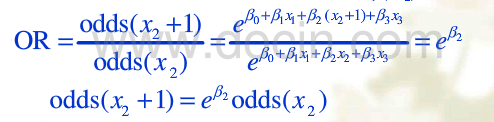当存在交互效应时：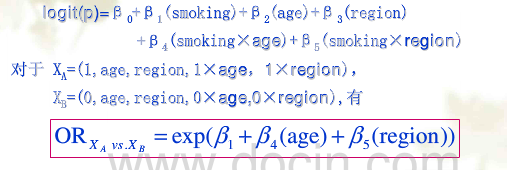logistic回归模型的参数估计与统计检验
参数估计（极大似然估计法）
似然函数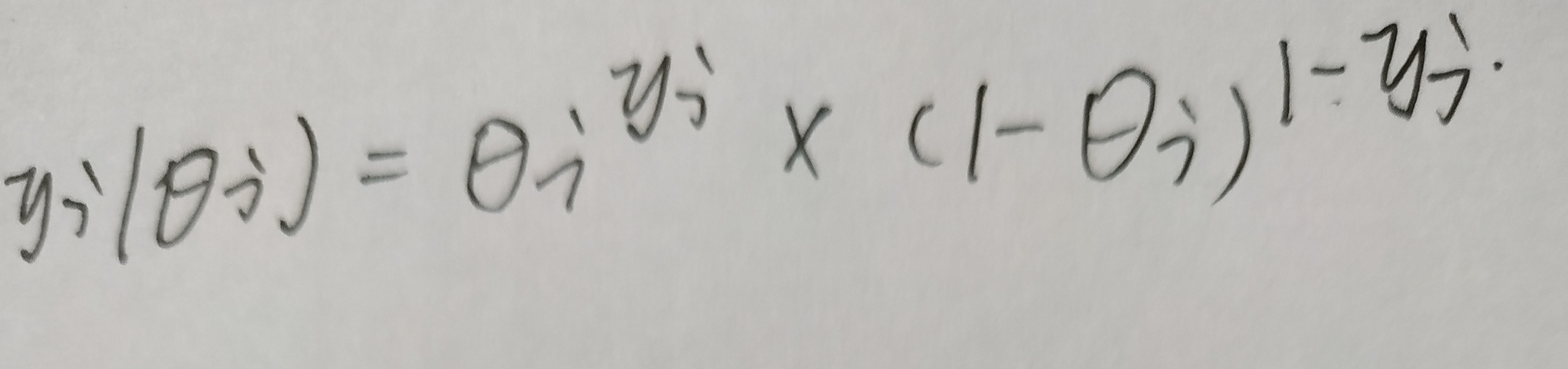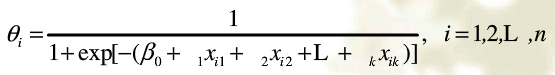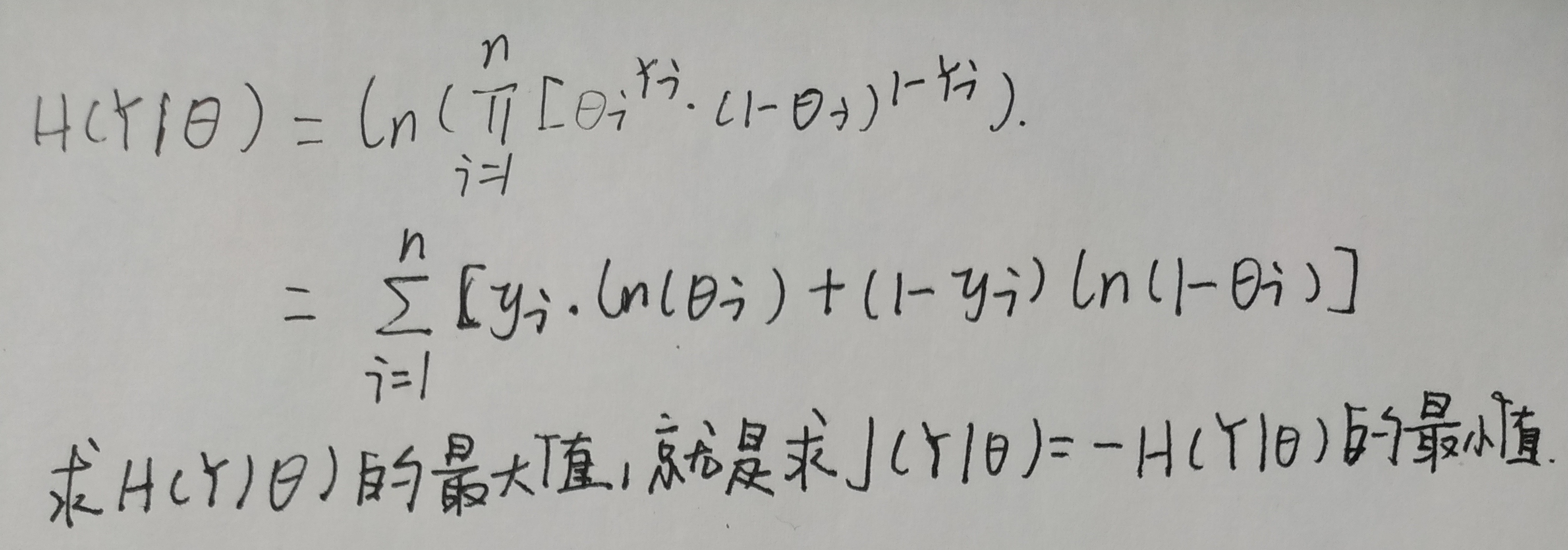梯度下降法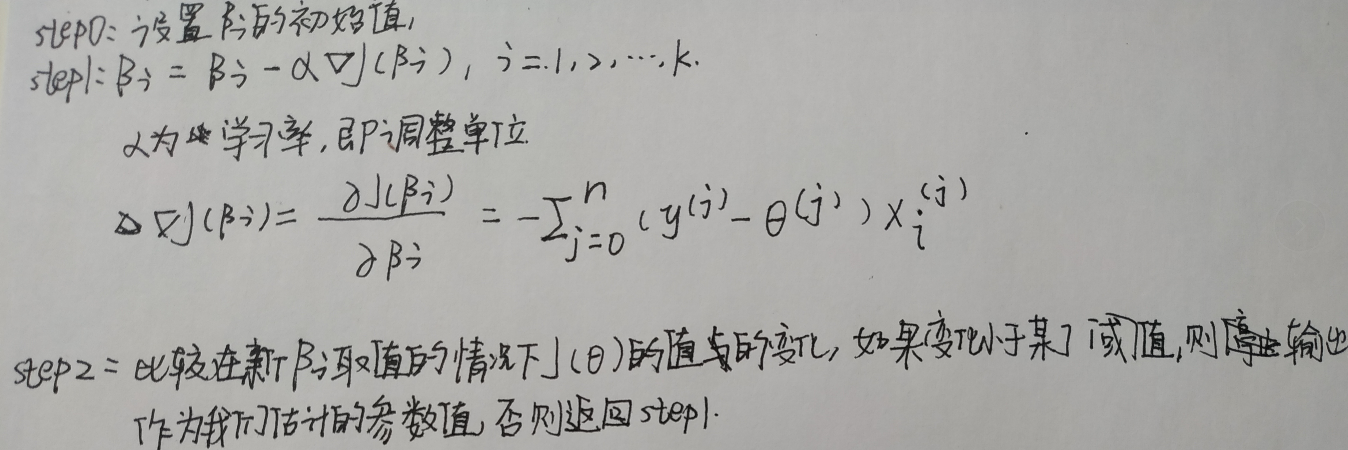统计检验
整体显著性检验
（1）-2 Log Likehood（-2LL）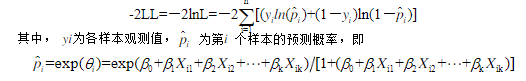-2LL越小，说明模型拟合得越好
（2）似然比L0/L的卡方检验
L0是解释变量均未引入回归方程前的似然函数值
L是解释变量引入回归方程后的似然函数值
H0：L0/L=1
-ln(L0/L)^2近似服从卡方分布
回归系数的显著性检验
（1）wald统计量检测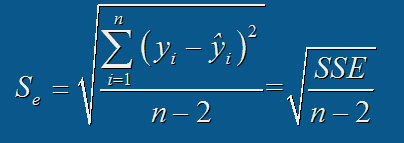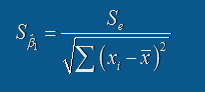H0：βi=0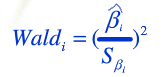wald检验统计量服从自由度为1的卡方分布
wald统计量的缺点：a. 受自变量间多重共线性的影响
b. 如果回归系数绝对值较大，标准误差也会扩大，导致wald检验统计量观测值偏小，不易拒绝零假设
（2）另一种方法
当回归系数绝对值较大时，可以对比较包含该变量和不包含该变量的模型的似然比变化，通过似然比卡方检验来检验该变量的回归系数不为零是否显著。
模型预测能力评价
混淆矩阵

Positive
Negative

Positive
TP
FN

Negative
FP
TN

简单评价指标
敏感性（sensitivity）召回率(recall)=TP/(TP+FN)
精确率（precision）=TP/（TP+FP）
准确率（accuracy）=（TP+TN）/（P+N）
特异度（specificity）=TN/(TN+FP)
ROC 曲线
真阳性率=TP/(TP+FN)
假阳性率=FP/(FP+TN)
以假阳性率为横轴，以真阳性率为纵轴，真阳性率和假阳性率会随着正例概率判定阈值的变化而变化，那么不同的阈值就会产生不同的真阳性率和假阳性率，即对应着坐标系中不同的点，将这些点连接起来就绘制好了ROC曲线。
ROC曲线下覆盖的面积越大，则模型的预测能力越高，因为面积越大说明在低阳性率的情况下真阳性率就可以达到较高水平，进一步说明正例的预测发生概率总体相对大于负例的预测发生概率。
正则化
目的：解决过拟合问题
代价函数J加入正则项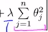放置模型参数过大
多分类Logistic回归模型
选择其中一个类作为参照类，建立k-1个双分类Logistic回归模型表示出其他各类的发生概论和参照类发生概率的比值，进而计算出各类实际发生概率，然后通过极大似然估计法估计各参数值。


展开全文机器学习 分类算法
• CNTK-Logistic回归模型 (CNTK - Logistic Regression Model) Advertisements 广告 Previous Page 上一页 Next Page 下一页 This chapter deals with constructing a logistic regression model in ...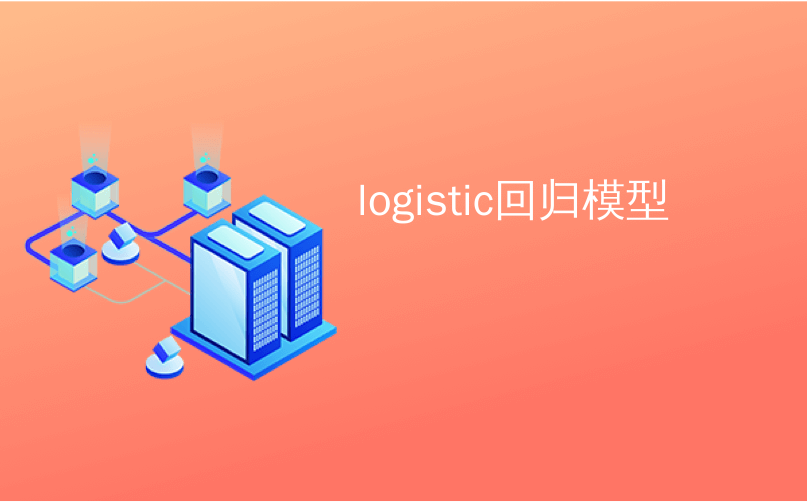logistic回归模型 CNTK-Logistic回归模型 (CNTK - Logistic Regression Model)
This chapter deals with constructing a logistic regression model in CNTK.
本章涉及在CNTK中构建逻辑回归模型。
Logistic回归模型的基础 (Basics of Logistic Regression model)
Logistic Regression, one of the simplest ML techniques, is a technique especially for binary classification. In other words, to create a prediction model in situations where the value of the variable to predict can be one of just two categorical values. One of the simplest examples of Logistic Regression is to predict whether the person is male or female, based on person’s age, voice, hairs and so on.
Logistic回归是最简单的ML技术之一，是一种专门用于二进制分类的技术。 换句话说，在预测变量的值可能只是两个分类值之一的情况下创建预测模型。 Logistic回归的最简单例子之一是根据人的年龄，声音，头发等来预测该人是男性还是女性。
例 (Example)
Let’s understand the concept of Logistic Regression mathematically with the help of another example −
让我们借助另一个示例在数学上理解Logistic回归的概念-
Suppose, we want to predict the credit worthiness of a loan application; 0 means reject, and 1 means approve, based on applicant debt , income and credit rating. We represent debt with X1, income with X2 and credit rating with X3.
假设我们要预测贷款申请的信用价值； 根据申请人的债务，收入和信用等级 ， 0表示拒绝，1表示批准。 我们用X1表示债务，用X2表示收入，用X3表示信用等级。
In Logistic Regression, we determine a weight value, represented by w, for every feature and a single bias value, represented by b.
在Logistic回归中，我们为每个特征确定一个权重值(用w表示)和一个偏置值(以b表示)。
Now suppose,
现在假设

X1 = 3.0
X2 = -2.0
X3 = 1.0

And suppose we determine weight and bias as follows −
并假设我们按以下方式确定权重和偏差-

W1 = 0.65, W2 = 1.75, W3 = 2.05 and b = 0.33

Now, for predicting the class, we need to apply the following formula −
现在，为了预测类别，我们需要应用以下公式-

Z = (X1*W1)+(X2*W2)+(X3+W3)+b
i.e. Z = (3.0)*(0.65) + (-2.0)*(1.75) + (1.0)*(2.05) + 0.33
= 0.83

Next, we need to compute P = 1.0/(1.0 + exp(-Z)). Here, the exp() function is Euler’s number.
接下来，我们需要计算P = 1.0 /(1.0 + exp(-Z)) 。 在这里，exp()函数是欧拉数。

P = 1.0/(1.0 + exp(-0.83)
= 0.6963

The P value can be interpreted as the probability that the class is 1. If P < 0.5, the prediction is class = 0 else the prediction (P >= 0.5) is class = 1.
P值可以解释为类别为1的概率。如果P <0.5，则预测为类别= 0，否则，预测(P> = 0.5)为类别= 1。
To determine the values of weight and bias, we must obtain a set of training data having the known input predictor values and known correct class labels values. After that, we can use an algorithm, generally Gradient Descent, in order to find the values of weight and bias.
要确定权重和偏倚的值，我们必须获得一组训练数据，该训练数据应具有已知的输入预测值和已知的正确类别标签值。 之后，我们可以使用一种算法(通常是“梯度下降”)来找到权重和偏差的值。
LR模型的实现实例 (LR model implementation example)
For this LR model, we are going to use the following data set −
对于此LR模型，我们将使用以下数据集-

1.0, 2.0, 0
3.0, 4.0, 0
5.0, 2.0, 0
6.0, 3.0, 0
8.0, 1.0, 0
9.0, 2.0, 0
1.0, 4.0, 1
2.0, 5.0, 1
4.0, 6.0, 1
6.0, 5.0, 1
7.0, 3.0, 1
8.0, 5.0, 1

To start this LR model implementation in CNTK, we need to first import the following packages −
要在CNTK中启动此LR模型实现，我们需要首先导入以下软件包-

import numpy as np
import cntk as C

The program is structured with main() function as follows −
该程序由main()函数构成，如下所示：

def main():
print("Using CNTK version = " + str(C.__version__) + "\n")

Now, we need to load the training data into memory as follows −
现在，我们需要按如下方式将训练数据加载到内存中：

data_file = ".\\dataLRmodel.txt"
print("Loading data from " + data_file + "\n")
features_mat = np.loadtxt(data_file, dtype=np.float32, delimiter=",", skiprows=0, usecols=[0,1])
labels_mat = np.loadtxt(data_file, dtype=np.float32, delimiter=",", skiprows=0, usecols=, ndmin=2)

Now, we will be creating a training program that creates a logistic regression model which is compatible with the training data −
现在，我们将创建一个训练程序，该程序将创建与训练数据兼容的逻辑回归模型-

features_dim = 2
labels_dim = 1
X = C.ops.input_variable(features_dim, np.float32)
y = C.input_variable(labels_dim, np.float32)
W = C.parameter(shape=(features_dim, 1)) # trainable cntk.Parameter
b = C.parameter(shape=(labels_dim))
z = C.times(X, W) + b
p = 1.0 / (1.0 + C.exp(-z))
model = p

Now, we need to create Lerner and trainer as follows −
现在，我们需要创建Lerner和Trainer，如下所示：

ce_error = C.binary_cross_entropy(model, y) # CE a bit more principled for LR
fixed_lr = 0.010
learner = C.sgd(model.parameters, fixed_lr)
trainer = C.Trainer(model, (ce_error), [learner])
max_iterations = 4000

LR模型训练 (LR Model training)
Once, we have created the LR model, next, it is time to start the training process −
一次，我们创建了LR模型，接下来，是时候开始训练过程了-

np.random.seed(4)
N = len(features_mat)
for i in range(0, max_iterations):
row = np.random.choice(N,1) # pick a random row from training items
trainer.train_minibatch({ X: features_mat[row], y: labels_mat[row] })
if i % 1000 == 0 and i > 0:
mcee = trainer.previous_minibatch_loss_average
print(str(i) + " Cross-entropy error on curr item = %0.4f " % mcee)

Now, with the help of the following code, we can print the model weights and bias −
现在，借助以下代码，我们可以打印模型权重和偏差-

np.set_printoptions(precision=4, suppress=True)
print("Model weights: ")
print(W.value)
print("Model bias:")
print(b.value)
print("")
if __name__ == "__main__":
main()

训练Logistic回归模型-完整示例 (Training a Logistic Regression model - Complete example)

import numpy as np
import cntk as C
def main():
print("Using CNTK version = " + str(C.__version__) + "\n")
data_file = ".\\dataLRmodel.txt" # provide the name and the location of data file
print("Loading data from " + data_file + "\n")
features_mat = np.loadtxt(data_file, dtype=np.float32, delimiter=",", skiprows=0, usecols=[0,1])
labels_mat = np.loadtxt(data_file, dtype=np.float32, delimiter=",", skiprows=0, usecols=, ndmin=2)
features_dim = 2
labels_dim = 1
X = C.ops.input_variable(features_dim, np.float32)
y = C.input_variable(labels_dim, np.float32)
W = C.parameter(shape=(features_dim, 1)) # trainable cntk.Parameter
b = C.parameter(shape=(labels_dim))
z = C.times(X, W) + b
p = 1.0 / (1.0 + C.exp(-z))
model = p
ce_error = C.binary_cross_entropy(model, y) # CE a bit more principled for LR
fixed_lr = 0.010
learner = C.sgd(model.parameters, fixed_lr)
trainer = C.Trainer(model, (ce_error), [learner])
max_iterations = 4000
np.random.seed(4)
N = len(features_mat)
for i in range(0, max_iterations):
row = np.random.choice(N,1) # pick a random row from training items
trainer.train_minibatch({ X: features_mat[row], y: labels_mat[row] })
if i % 1000 == 0 and i > 0:
mcee = trainer.previous_minibatch_loss_average
print(str(i) + " Cross-entropy error on curr item = %0.4f " % mcee)
np.set_printoptions(precision=4, suppress=True)
print("Model weights: ")
print(W.value)
print("Model bias:")
print(b.value)
if __name__ == "__main__":
main()

输出量 (Output)

Using CNTK version = 2.7
1000 cross entropy error on curr item = 0.1941
2000 cross entropy error on curr item = 0.1746
3000 cross entropy error on curr item = 0.0563
Model weights:
[-0.2049]
[0.9666]]
Model bias:
[-2.2846]

使用经过训练的LR模型进行预测 (Prediction using trained LR Model)
Once the LR model has been trained, we can use it for prediction as follows −
一旦训练了LR模型，我们就可以将其用于预测，如下所示：
First of all, our evaluation program imports the numpy package and loads the training data into a feature matrix and a class label matrix in the same way as the training program we implement above −
首先，我们的评估程序导入numpy程序包，并将训练数据加载到特征矩阵和类标签矩阵中，方法与我们在上面实现的训练程序相同-

import numpy as np
def main():
data_file = ".\\dataLRmodel.txt" # provide the name and the location of data file
features_mat = np.loadtxt(data_file, dtype=np.float32, delimiter=",",
skiprows=0, usecols=(0,1))
labels_mat = np.loadtxt(data_file, dtype=np.float32, delimiter=",",
skiprows=0, usecols=, ndmin=2)

Next, it is time to set the values of the weights and the bias that were determined by our training program −
接下来，是时候设置由我们的训练计划确定的权重和偏差的值了-

print("Setting weights and bias values \n")
weights = np.array([0.0925, 1.1722], dtype=np.float32)
bias = np.array([-4.5400], dtype=np.float32)
N = len(features_mat)
features_dim = 2

Next our evaluation program will compute the logistic regression probability by walking through each training items as follows −
接下来，我们的评估程序将通过遍历每个训练项目来计算逻辑回归概率，如下所示：

print("item pred_prob pred_label act_label result")
for i in range(0, N): # each item
x = features_mat[i]
z = 0.0
for j in range(0, features_dim):
z += x[j] * weights[j]
z += bias
pred_prob = 1.0 / (1.0 + np.exp(-z))
pred_label = 0 if pred_prob < 0.5 else 1
act_label = labels_mat[i]
pred_str = ‘correct’ if np.absolute(pred_label - act_label) < 1.0e-5 \
else ‘WRONG’
print("%2d %0.4f %0.0f %0.0f %s" % \ (i, pred_prob, pred_label, act_label, pred_str))

Now let us demonstrate how to do prediction −
现在让我们演示如何进行预测-

x = np.array([9.5, 4.5], dtype=np.float32)
print("\nPredicting class for age, education = ")
print(x)
z = 0.0
for j in range(0, features_dim):
z += x[j] * weights[j]
z += bias
p = 1.0 / (1.0 + np.exp(-z))
print("Predicted p = " + str(p))
if p < 0.5: print("Predicted class = 0")
else: print("Predicted class = 1")

完整的预测评估程序 (Complete prediction evaluation program)

import numpy as np
def main():
data_file = ".\\dataLRmodel.txt" # provide the name and the location of data file
features_mat = np.loadtxt(data_file, dtype=np.float32, delimiter=",",
skiprows=0, usecols=(0,1))
labels_mat = np.loadtxt(data_file, dtype=np.float32, delimiter=",",
skiprows=0, usecols=, ndmin=2)
print("Setting weights and bias values \n")
weights = np.array([0.0925, 1.1722], dtype=np.float32)
bias = np.array([-4.5400], dtype=np.float32)
N = len(features_mat)
features_dim = 2
print("item pred_prob pred_label act_label result")
for i in range(0, N): # each item
x = features_mat[i]
z = 0.0
for j in range(0, features_dim):
z += x[j] * weights[j]
z += bias
pred_prob = 1.0 / (1.0 + np.exp(-z))
pred_label = 0 if pred_prob < 0.5 else 1
act_label = labels_mat[i]
pred_str = ‘correct’ if np.absolute(pred_label - act_label) < 1.0e-5 \
else ‘WRONG’
print("%2d %0.4f %0.0f %0.0f %s" % \ (i, pred_prob, pred_label, act_label, pred_str))
x = np.array([9.5, 4.5], dtype=np.float32)
print("\nPredicting class for age, education = ")
print(x)
z = 0.0
for j in range(0, features_dim):
z += x[j] * weights[j]
z += bias
p = 1.0 / (1.0 + np.exp(-z))
print("Predicted p = " + str(p))
if p < 0.5: print("Predicted class = 0")
else: print("Predicted class = 1")
if __name__ == "__main__":
main()

输出量 (Output)
Setting weights and bias values.
设置权重和偏差值。

Item  pred_prob  pred_label  act_label  result
0   0.3640         0             0     correct
1   0.7254         1             0      WRONG
2   0.2019         0             0     correct
3   0.3562         0             0     correct
4   0.0493         0             0     correct
5   0.1005         0             0     correct
6   0.7892         1             1     correct
7   0.8564         1             1     correct
8   0.9654         1             1     correct
9   0.7587         1             1     correct
10  0.3040         0             1      WRONG
11  0.7129         1             1     correct
Predicting class for age, education =
[9.5 4.5]
Predicting p = 0.526487952
Predicting class = 1

翻译自: https://www.tutorialspoint.com/microsoft_cognitive_toolkit/microsoft_cognitive_toolkit_logistic_regression_model.htmlogistic回归模型
展开全文python 机器学习 深度学习 算法 人工智能
• 文章目录Pytorh Note11 Logistic 回归模型Logistic 回归模型模型形式Sigmoid 函数回归问题 vs 分类问题损失函数Logistic 例子定义Logistic 回归模型训练模型 Training拟合曲线 和 结果展示 全部笔记的汇总贴：...
Pytorch Note11 Logistic 回归模型
文章目录Pytorch Note11 Logistic 回归模型Logistic 回归模型模型形式Sigmoid 函数回归问题 vs 分类问题损失函数Logistic 例子定义Logistic 回归模型训练模型 Training拟合曲线 和 结果展示
全部笔记的汇总贴：Pytorch Note 快乐星球
Logistic 回归模型
机器学习中的监督学习主要分为回归问题和分类问题，我们之前已经讲过回归问题了，它希望预测的结果是连续的，那么分类问题所预测的结果就是离散的类别。这时输入变量可以是离散的，也可以是连续的，而监督学习从数据中学习一个分类模型或者分类决策函数，它被称为分类器（classifier）。分类器对新的输入进行输出预测，这个过程即称为分类（classification）。例如，判断邮件是否为垃圾邮件，医生判断病人是否生病，或者预测明天天气是否下雨等。同时分类问题中包括有二分类和多分类问题，我们下面先讲一下最著名的二分类算法——Logistic 回归。首先从 Logistic 回归的起源说起。
Logistic 起源于对人口数量增长情况的研究，后来又被应用到了对于微生物生长情况的研究，以及解决经济学相关的问题，现在作为回归分析的一个分支来处理分类问题，先从 Logistic 分布入手，再由 Logistic 分布推出 Logistic 回归
模型形式
Logistic 回归的模型形式和线性回归一样，都是 $y = wx + b$，其中 x 可以是一个多维的特征，唯一不同的地方在于 Logistic 回归会对 y 作用一个 logistic 函数，将其变为一种概率的结果。 Logistic 函数作为 Logistic 回归的核心，我们下面讲一讲 Logistic 函数，也被称为 Sigmoid 函数。
Sigmoid 函数
Sigmoid 函数非常简单，其公式如下
$f(x) = \frac{1}{1 + e^{-x}}$
Sigmoid 函数的图像如下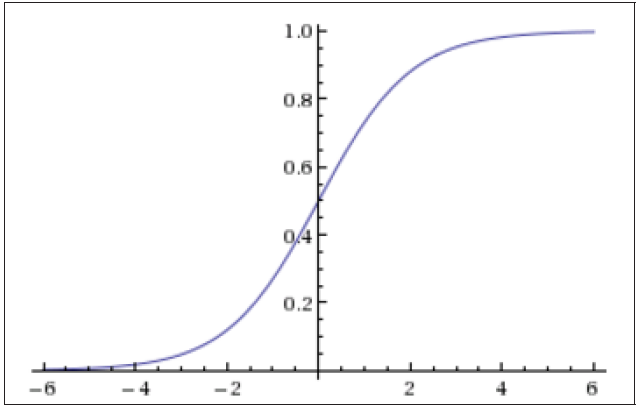可以看到 Sigmoid 函数的范围是在 0 ~ 1 之间，所以任何一个值经过了 Sigmoid 函数的作用，都会变成 0 ~ 1 之间的一个值，这个值可以形象地理解为一个概率，比如对于二分类问题，这个值越小就表示属于第一类，这个值越大就表示属于第二类。
另外一个 Logistic 回归的前提是确保你的数据具有非常良好的线性可分性，也就是说，你的数据集能够在一定的维度上被分为两个部分，比如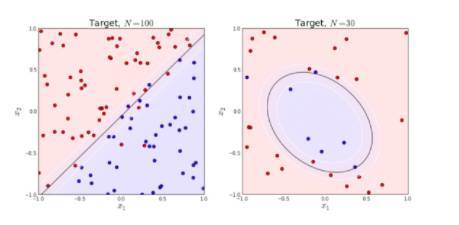可以看到，上面红色的点和蓝色的点能够几乎被一个平面分割开来
回归问题 vs 分类问题
Logistic 回归处理的是一个分类问题，而上一个模型是回归模型，那么回归问题和分类问题的区别在哪里呢？
从上面的图可以看出，分类问题希望把数据集分到某一类，比如一个 3 分类问题，那么对于任何一个数据点，我们都希望找到其到底属于哪一类，最终的结果只有三种情况，{0, 1, 2}，所以这是一个离散的问题。
而回归问题是一个连续的问题，比如曲线的拟合，我们可以拟合任意的函数结果，这个结果是一个连续的值。
分类问题和回归问题是机器学习和深度学习的第一步，拿到任何一个问题，我们都需要先确定其到底是分类还是回归，然后再进行算法设计
损失函数
前一节对于回归问题，我们有一个 loss 去衡量误差，那么对于分类问题，我们如何去衡量这个误差，并设计 loss 函数呢？
Logistic 回归使用了 Sigmoid 函数将结果变到 0 ~ 1 之间，对于任意输入一个数据，经过 Sigmoid 之后的结果我们记为 $\hat{y}$，表示这个数据点属于第二类的概率，那么其属于第一类的概率就是 $1-\hat{y}$。如果这个数据点属于第二类，我们希望 $\hat{y}$ 越大越好，也就是越靠近 1 越好，如果这个数据属于第一类，那么我们希望 $1-\hat{y}$ 越大越好，也就是 $\hat{y}$ 越小越好，越靠近 0 越好，所以我们可以这样设计我们的 loss 函数
$loss = -(y * log(\hat{y}) + (1 - y) * log(1 - \hat{y}))$
其中 y 表示真实的 label，只能取 {0, 1} 这两个值，因为 $\hat{y}$ 表示经过 Logistic 回归预测之后的结果，是一个 0 ~ 1 之间的小数。如果 y 是 0，表示该数据属于第一类，我们希望 $\hat{y}$ 越小越好，上面的 loss 函数变为
$loss = - (log(1 - \hat{y}))$
在训练模型的时候我们希望最小化 loss 函数，根据 log 函数的单调性，也就是最小化 $\hat{y}$，与我们的要求是一致的。
而如果 y 是 1，表示该数据属于第二类，我们希望 $\hat{y}$ 越大越好，同时上面的 loss 函数变为
$loss = -(log(\hat{y}))$
我们希望最小化 loss 函数也就是最大化 $\hat{y}$，这也与我们的要求一致。
所以通过上面的论述，说明了这么构建 loss 函数是合理的。
Logistic 例子
import torch
from torch.autograd import Variable
import numpy as np
import matplotlib.pyplot as plt
%matplotlib inline

我们从 data.txt 读入数据，感兴趣的同学可以打开 data.txt 文件进行查看
读入数据点之后我们根据不同的 label 将数据点分为了红色和蓝色，并且画图展示出来了
# 从 data.txt 中读入点
with open('./data.txt', 'r') as f:
data_list = [i.split('\n').split(',') for i in f.readlines()]
data = [(float(i), float(i), float(i)) for i in data_list]

# 标准化
x0_max = max([i for i in data])
x1_max = max([i for i in data])
data = [(i/x0_max, i/x1_max, i) for i in data]

x0 = list(filter(lambda x: x[-1] == 0.0, data)) # 选择第一类的点
x1 = list(filter(lambda x: x[-1] == 1.0, data)) # 选择第二类的点

plot_x0 = [i for i in x0]
plot_y0 = [i for i in x0]
plot_x1 = [i for i in x1]
plot_y1 = [i for i in x1]

plt.plot(plot_x0, plot_y0, 'ro', label='x_0')
plt.plot(plot_x1, plot_y1, 'bo', label='x_1')
plt.legend(loc='best')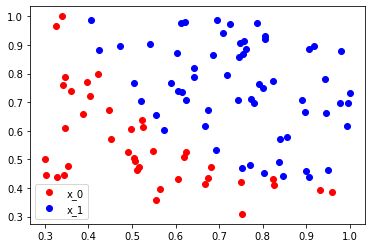定义Logistic 回归模型
class  LogisticRegression(nn.Module):
def  __init__(self):
super(LogisticRegression,  self).__init__()
self.lr  =  nn.Linear(2,  1)
self.sm  =  nn.Sigmoid()

def  forward(self,  x):
x  =  self.lr(x)
x  =  self.sm(x)
return  x

logistic_model  =  LogisticRegression()
if  torch.cuda.is_available():
logistic_model.cuda()

criterion  =  nn.BCELoss()
optimizer  =  torch.optim.SGD(logistic_model.parameters(),  lr=1e-3,
momentum=0.9)

这里  nn.BCELoss 是二分类的损失函数，torch.optim.SGD 是随机梯度下降优化函数。然后训练模型，并且间隔一定的迭代次数输出结果。
训练模型 Training
for  epoch  in  range(50000):
if  torch.cuda.is_available():
x  =  Variable(x_data).cuda()
y  =  Variable(y_data).cuda()
else:
x  =  Variable(x_data)
y  =  Variable(y_data)
#  ==================forward==================
out  =  logistic_model(x)
loss  =  criterion(out,  y)
print_loss  =  loss.data
mask  =  out.ge(0.5).float()
correct  =  (mask  ==  y).sum()
acc  =  correct.data  /  x.size(0)
#  ===================backward=================
optimizer.zero_grad()

loss.backward()
optimizer.step()
if  (epoch+1)  %  1000  ==  0:
print('*'*10)
print('epoch  {}'.format(epoch+1))
print('loss  is  {:.4f}'.format(print_loss))
print('acc  is  {:.4f}'.format(acc))

其中  mask=out.ge(0.5).float() 是判断输出结果如果大于 0.5 就等于 1，小于0.5 就等于 0，通过这个来计算模型分类的准确率。
因为数据相对简单，同时我们使用的是也是简单的线性 Logistic 回归，loss 已经降得相对较低，同时也有 91% 的准确率。
epoch  46000
loss  is  0.3116
acc  is  0.9100
**********
epoch  47000
loss  is  0.3098
acc  is  0.9100
**********
epoch  48000
loss  is  0.3080
acc  is  0.9100
**********
epoch  49000
loss  is  0.3063
acc  is  0.9100
**********
epoch  50000
loss  is  0.3046
acc  is  0.9100

拟合曲线 和 结果展示
w0,  w1  =  logistic_model.lr.weight
w0  =  w0.data.cpu()
w1  =  w1.data.cpu()
b  =  logistic_model.lr.bias.data.cpu()
plot_x  =  np.arange(0.2,  1,  0.1)

plot_y  =  (-w0  *  plot_x  -  b)  /  w1
plt.plot(plot_x, plot_y, 'g', label='cutting line')
plt.plot(plot_x0, plot_y0, 'ro', label='x_0')
plt.plot(plot_x1, plot_y1, 'bo', label='x_1')
plt.legend(loc='best')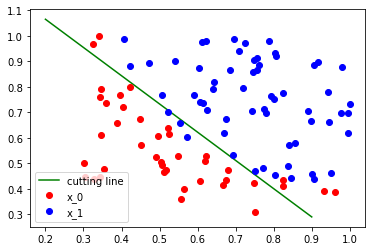以上我们介绍了分类问题中的二分类问题和 Logistic 回归算法，一般来说，Logistic回归也可以处理多分类问题，但最常见的还是应用在处理二分类问题上，下一节会讲一下如何用神经网络解决非线性的分类问题
下一章传送门：Note12 多层神经网络


展开全文python 机器学习 人工智能 深度学习 pytorch
• 本章介绍Logistic回归分类模型，并通过案例讲解如何使用Logistic回归模型人工智能学习 深度学习框架 深度学习算法 python深度学习
• 在这里讲述如何通过MATLAB的代码实现多元logistic回归模型，对于想用MATLAB来实现多元logistic回归模型的朋友有很大的帮助。
• ## logistic回归模型

千次阅读 2012-09-04 21:23:52
logistic regression是统计学习中...回归模型： 给定一个数据集合(x1,y1)(x2,y2)...(xn,yn)，有监督学习构建模型，学习过程就是模型参数θ的学习过程。作为discrimination algorithm，对 P(Y|X;θ)建模，分布式应用 dataset algorithm input 算法
• 本章介绍Logistic回归分类模型，并通过案例讲解如何使用Logistic回归模型机器学习 人工智能
• 本章介绍Logistic回归分类模型，并通过案例讲解如何使用Logistic回归模型机器学习 人工智能
• 基于Logistic回归模型的人口预测分析 尹东旭李 阳马雨晨 指导老师徐 慧 (空军工程大学西安 XXXXXX) 摘 要本文在数值微分法和最小二乘法曲线拟合的基础上对Logistic回归模型进行参数估计预测了人口城镇化和老龄化两个...
• 本章介绍Logistic回归分类模型，并通过案例讲解如何使用Logistic回归模型机器学习 人工智能
• 本章介绍Logistic回归分类模型，并通过案例讲解如何使用Logistic回归模型机器学习 人工智能
• Logistic回归模型下的一些岭参数性质，杨成敏，黎雅莲，这篇文章主要介绍基于极大似然（ML）估计Logistic岭回归（logistic ridge regression）模型（LRR）岭参数k的新方法。运用蒙特卡洛模拟研究分别
• Logistic回归模型学习总结第一：深度学习单层网络第二：Logistic回归模型概述2.1建立一个数学模型：把变量和结果表示出来——线性变换出来吧2.2 怎么把y值映射到0和1这两个数——非线性变换出来了第三：Logistic回归...
• 我们知道可以通过回归模型进行拟合，并挖掘各个特征变量对模板变量的影响机制，但是，对于分类问题，由于目标变量是离散型变量，因此，不能直接应用回归模型进行解决，这时，可以使用Logistic回归模型进行解决。...
• Logistic回归模型的拟合优度评估主题吸引了许多科学家和研究人员的关注。 拟合优度测试是确定拟合模型的适用性的方法。 在logistic回归模型中提出和讨论了许多评估拟合优度的方法，但是，拟合优度统计量的渐近分布...
• MATLAB源码集锦-基于Logistic回归模型评估企业还款能力代码
• 对实验结果进行Logistic回归建模，使用Logistic回归模型计算的工艺参数进行验证实验，样件内部未发现内部缺陷，说明Logistic回归模型结果与实际结果有较高的一致性。Logistic回归模型对激光金属直接成形工艺参数的...
• Logistic回归模型和判别分析方法都可以达到对样本分类的目的，比较和分析这2种方法的差异以及其各自的特点，可以为更好的应用提供参考。从类别表现、样本情况、归类函数、归类原则、预测功效等5个方面对Logistic回归...
• 由此可见logistic回归是从线性回归模型推广而来的，线性回归模型如下： -----------线性方程形式 ----------------向量形式 h(x)输出为连续的值，但是实际中会有"输出为离散型变量"这样的需求，比如给定...线性回归
• 上一篇文章介绍了线性判别模型，本文介绍线性生成模型——logistic回归模型。本文介绍logstic回归模型相关的知识，为了更好理解模型的决策边界函数，本文同时分析了多元变量的协方差对概率分布的影响。 目录 1、...
• 王济川 郭志强 Logistic回归模型——方法与应用 王济川 郭志强 Logistic回归模型——方法与应用 王济川 郭志强 Logistic回归模型——方法与应用
• logistic回归模型为： 对上面的模型进行变换，得到线性形式的logistic回归模型： 在二项分布族中，logistic回归是重要的模型。在某些回归问题中，响应变量是分类的，经常是要么成功，要么失败。 在R语言构建数据...
• ## 用R语言建立logistic回归模型

万次阅读 多人点赞 2019-02-20 22:13:32
用R语言建立logistic回归模型 公式：fm&lt;-glm(formula,family=binomial(link=logit),data=data.frame) 其中：link=logit可以不写。 函数 用途 summary() 展示拟合模型的详细结果 coefficients() 列......